## Transient transport problem - nonlinear case

 NlTransientTransportProblem nsteps #(in) deltaT #(rn)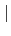deltaTfunction #(in) alpha #(rn) [initT #(rn)] [lumpedcapa #()] [nsmax #(in)] rtol #(rn) [manrmsteps #(in)] [sparselinsolverparams #(...)] [exportfields #(ia)] [changingProblemSize]

Implicit integration scheme for transient transport problems. The generalized midpoint rule (sometimes called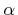-method) is used for time discretization, with alpha parameter, which has limits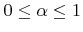. For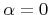explicit Euler forward method is obtained, for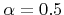implicit trapezoidal rule is recovered, which is unconditionally stable, second-order accurate in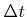, and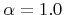yields implicit Euler backward method, which is unconditionally stable, and first-order accurate in. See matlibmanual.pdf for solution algorithm.

deltaT is time step length used for integration, nsteps parameter specifies number of time steps to be solved. For deltaTfunction and initT see section 3.2.2. Parameter maxiter determines the maximum number of iterations allowed to reach equilibrium (default is 30). Norms of residual physical quantity (heat, mass) described by solution vector and the change of solution vector are determined in each iteration. The convergence is reached, when the norms are less than the value given by rtol. If manrmsteps parameter is nonzero, then the modified N-R scheme is used, with the left-hand side matrix updated after manrmsteps steps. nsmax maximum number of iterations per time step, default is 30. If lumpedcapa is set, then the stabilization of numerical algorithm using lumped capacity matrix will be used, reducing the initial oscillations.

See the Section 3.2.1 for an explanation on exportfields. The meaning of changingProblemSize is given in Section 3.2.2.

Note: This problem type requires transport module and it can be used only when this module is included in your oofem configuration.

Borek Patzak
2018-01-02